# Quasi-norm

(diff) ← Older revision | Latest revision (diff) | Newer revision → (diff)
A non-negative function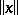defined on a linear spaceand satisfying the same axioms as a norm except for the triangle inequality, which is replaced by the weaker requirement: There exists a constant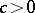such that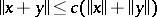for all.
The topology of a locally bounded topological vector space can be given by a quasi-norm. Conversely, a quasi-normed vector space is locally bounded. Here a setin a topological vector space is bounded if for each open neighbourhoodof zero there is a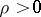such that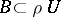, and a topological vector space is locally bounded if there is a bounded neighbourhood of zero. Given a circled bounded neighbourhoodof zero in a topological vector space(a set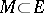is circled iffor all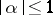), the Minkowski functional ofis defined by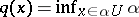. It is a quasi-norm.